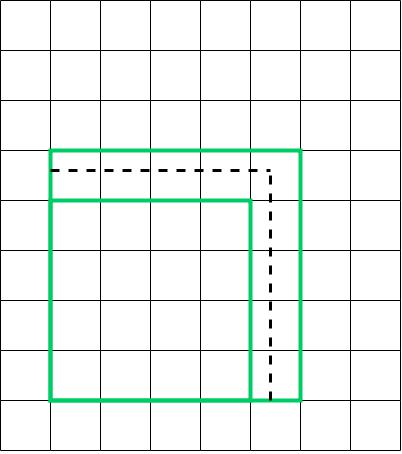# Determine square roots of rational numbers

### Determine square roots of rational numbers

In this section, we will look at how to evaluate a rational number by using square roots. We will also work on questions determining whether a rational number is a perfect square. We will also evaluate the square roots of rational numbers.

#### Lessons

• 1.
Use the graph below to determine a rational number with a square root between 4 and 5.• 2.
Use the side lengths below to estimate and calculate the area of each square.
a)
5.2 cm

b)
0.027 km

• 3.
Find out if each of the following rational numbers is a perfect square.
a)
$\frac{{81}}{{16}}$

b)
0.1

c)
0.01

d)
$\frac{5}{{14}}$

• 4.
Evaluate.
a)
$\sqrt {361}$

b)
$\sqrt {2209}$

c)
$\sqrt {0.0169}$

d)
$\sqrt {5.76}$

• 5.
Calculate.
a)
$\sqrt {56}$, to the nearest tenth

b)
$\sqrt {3.7}$, to the nearest hundredth

c)
$\sqrt {0.96}$ , to the nearest hundredth

d)
$\sqrt {0.066}$ , to the nearest hundredth